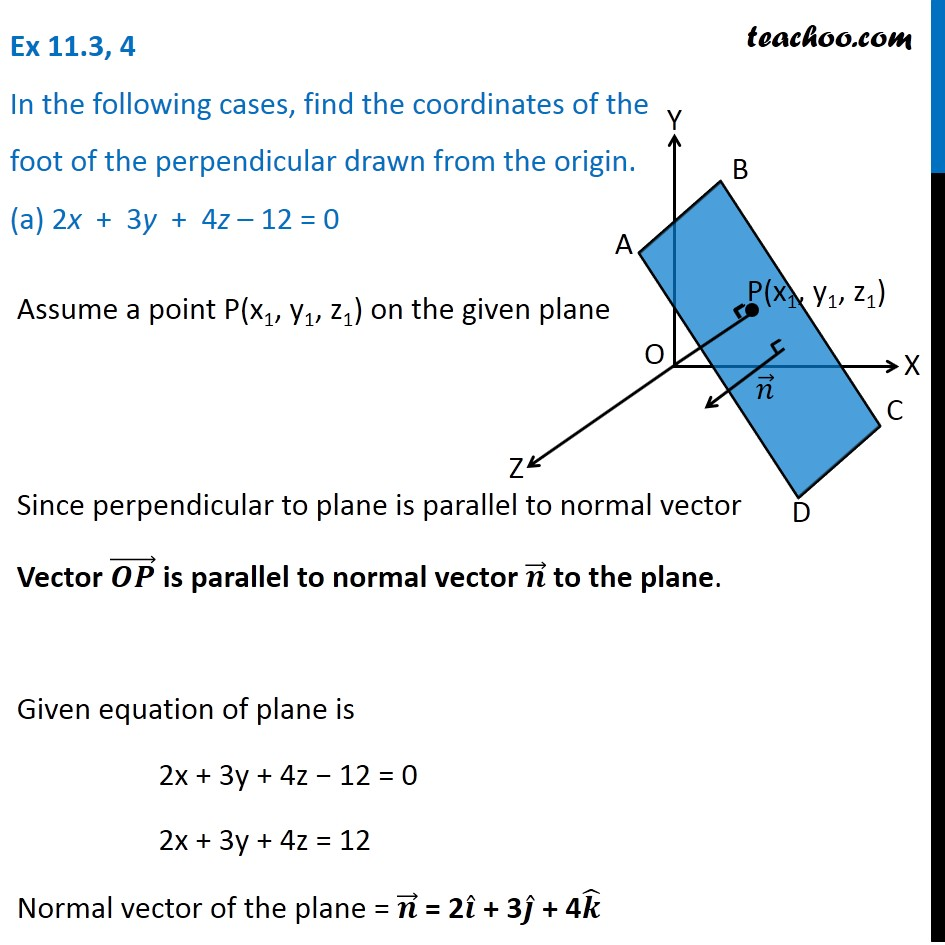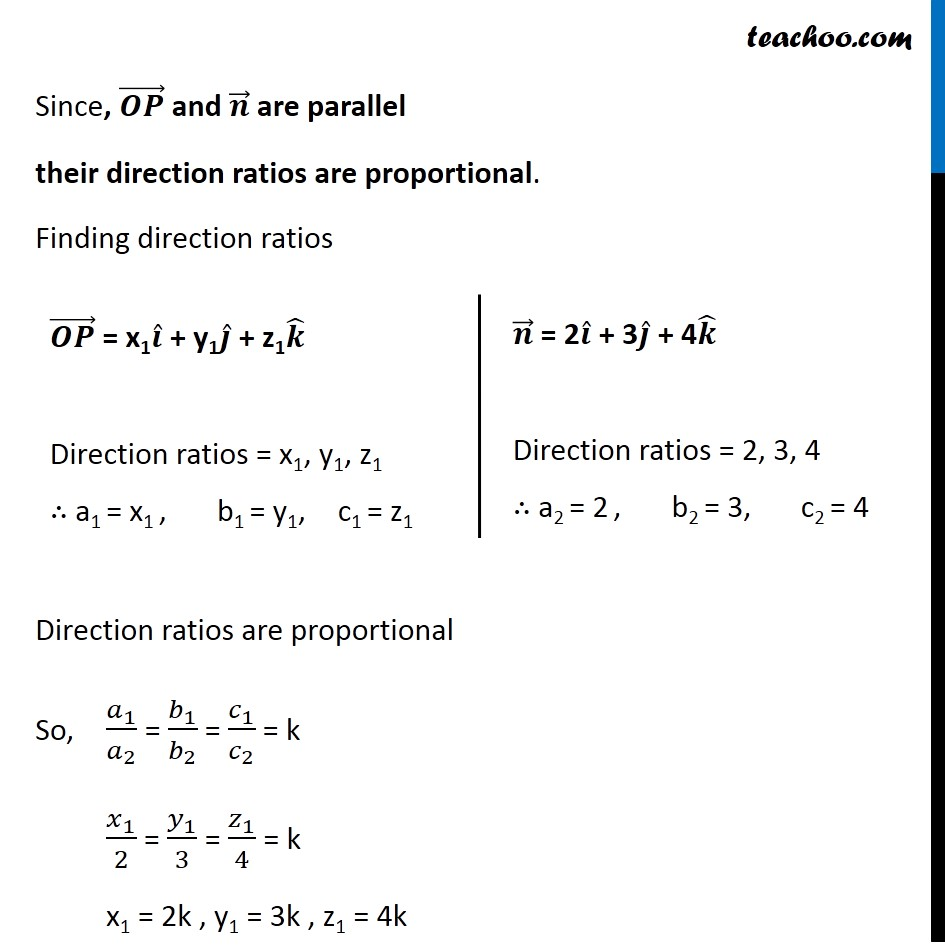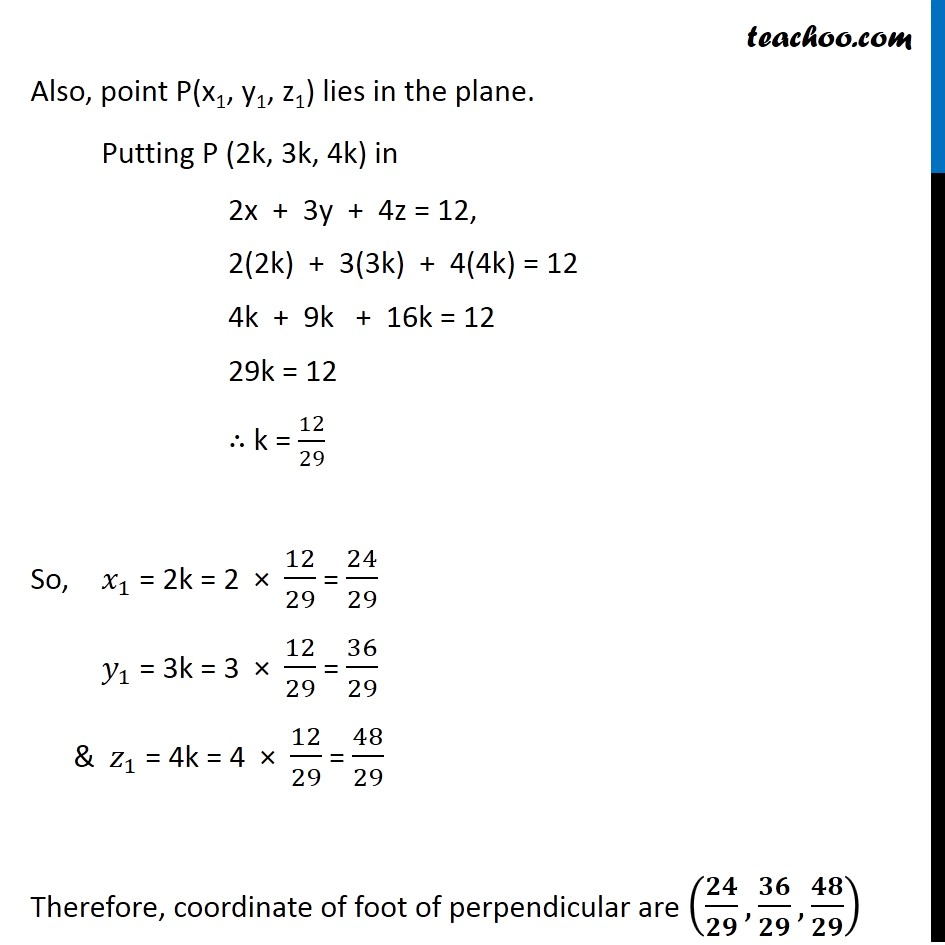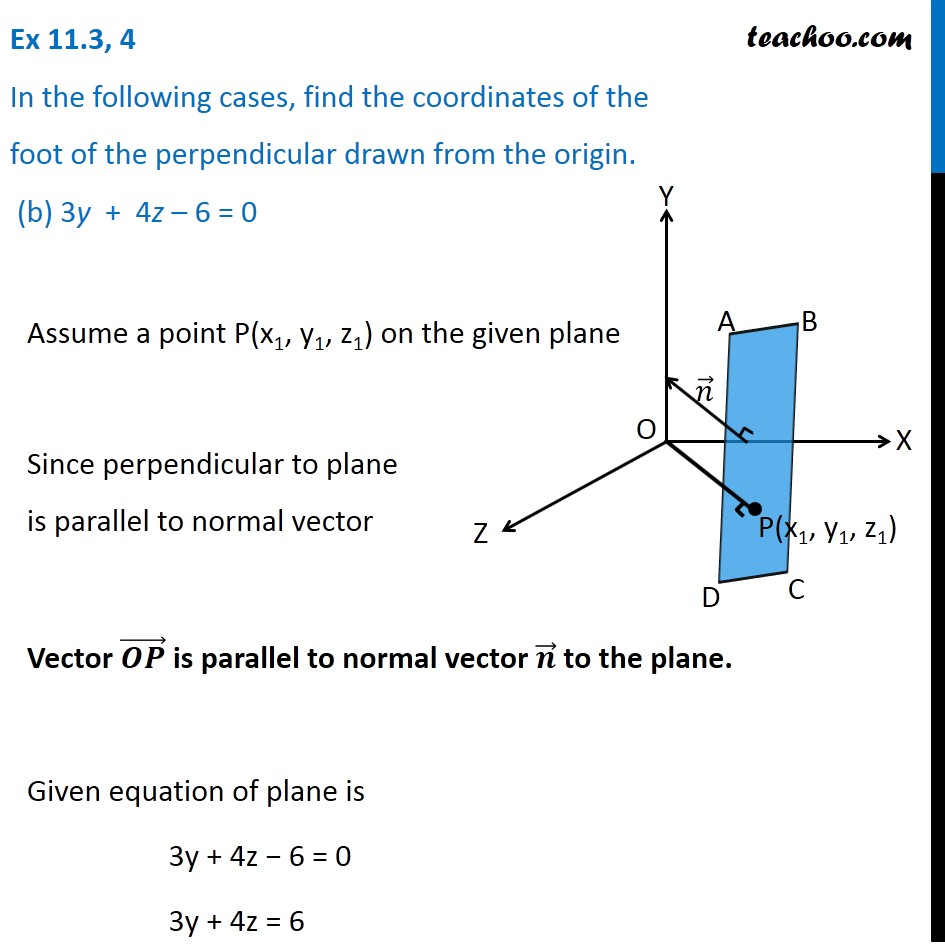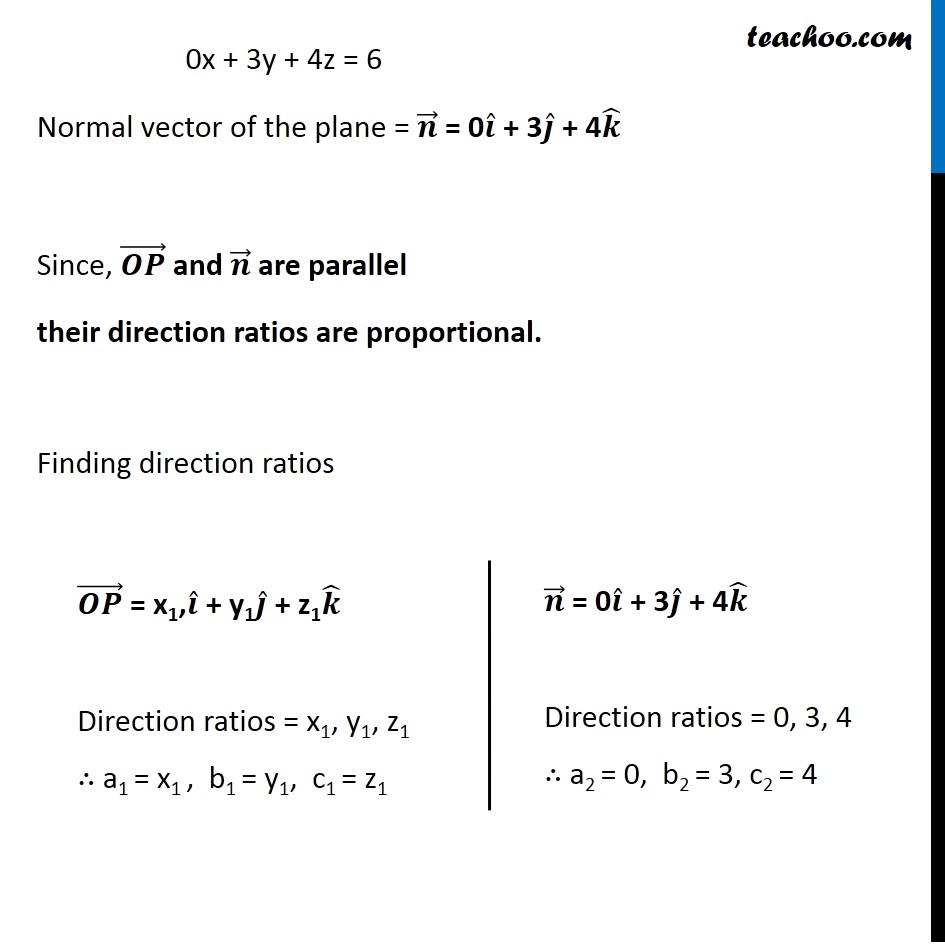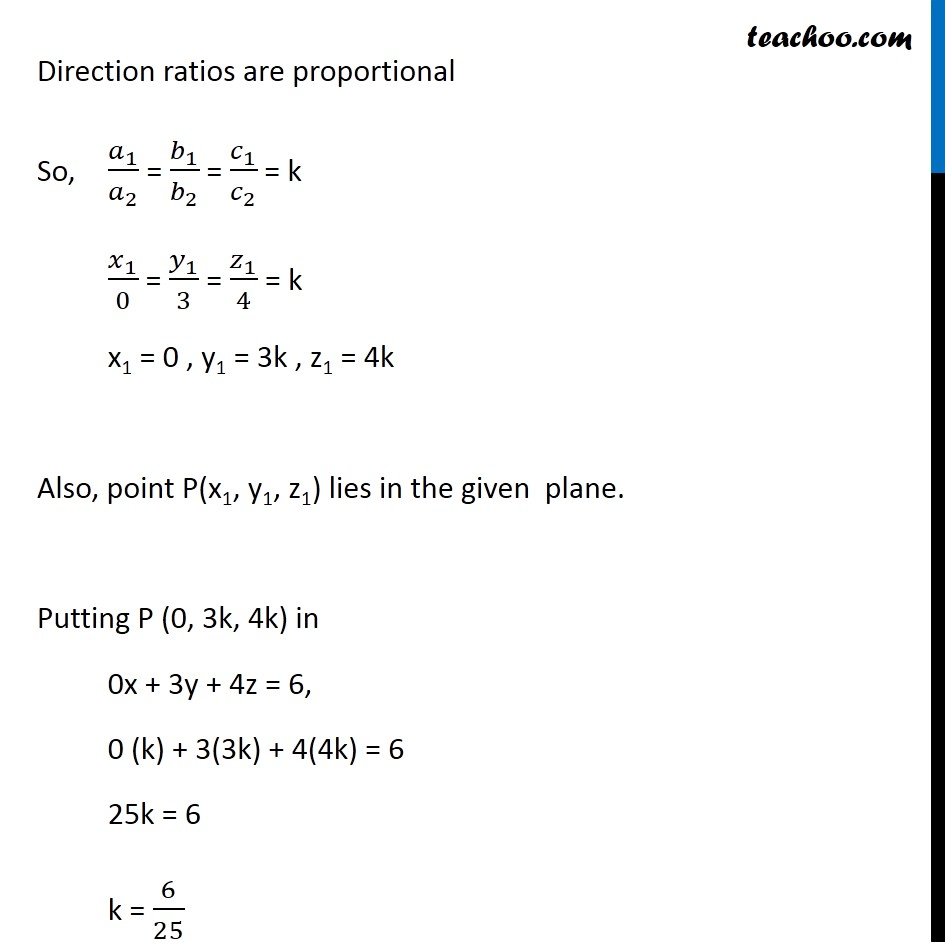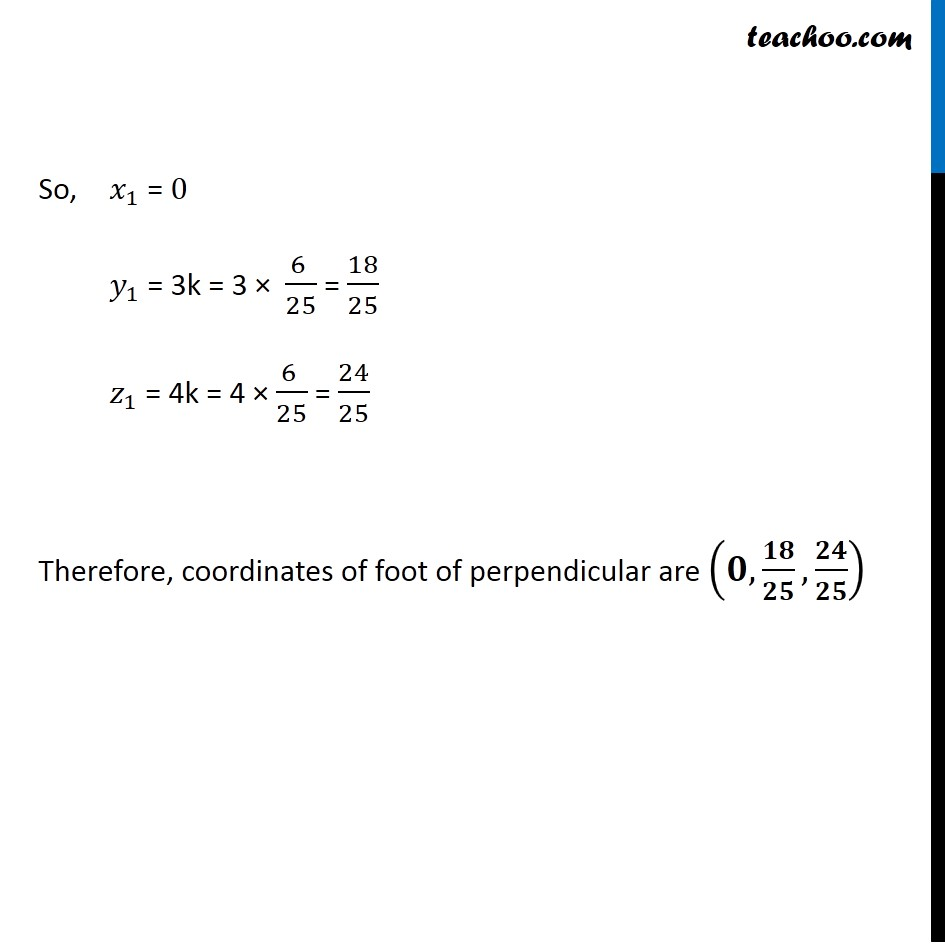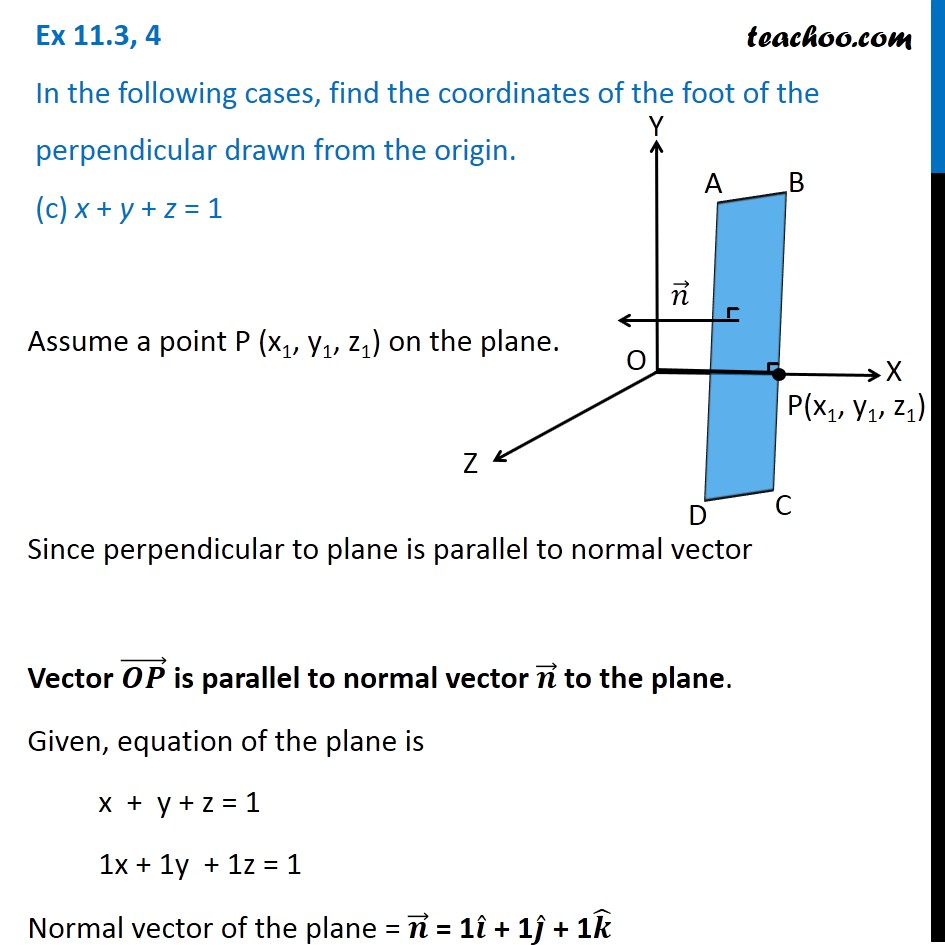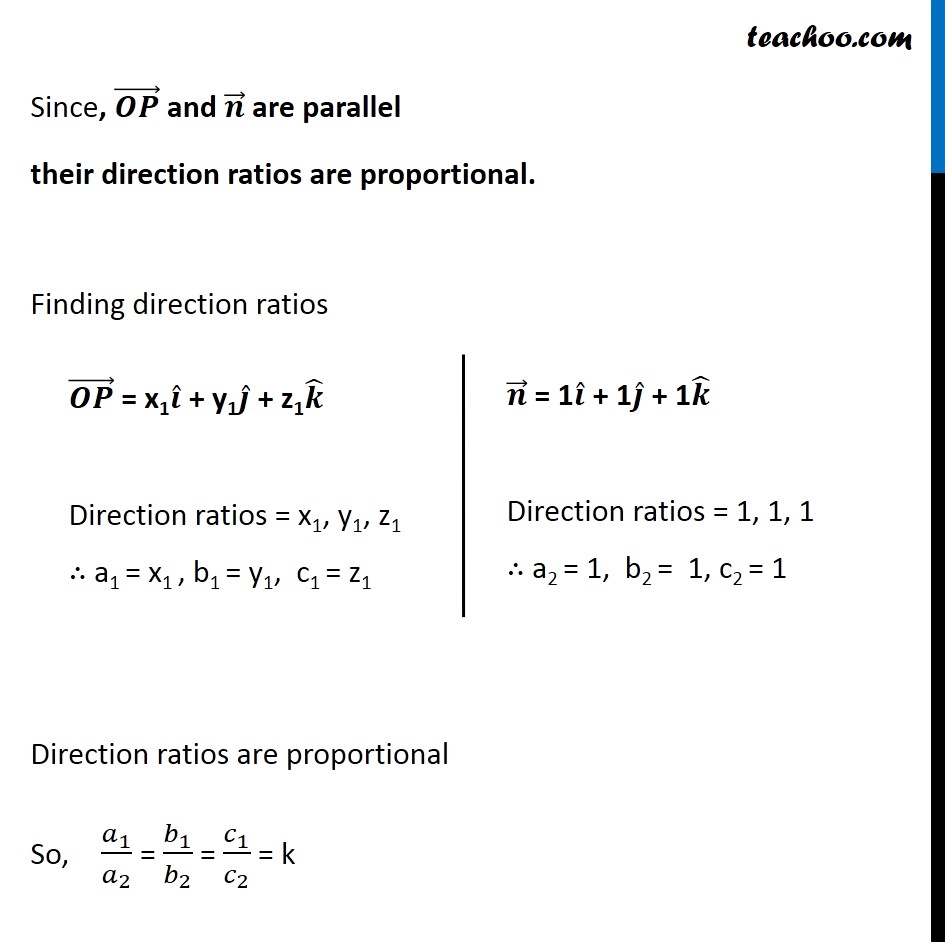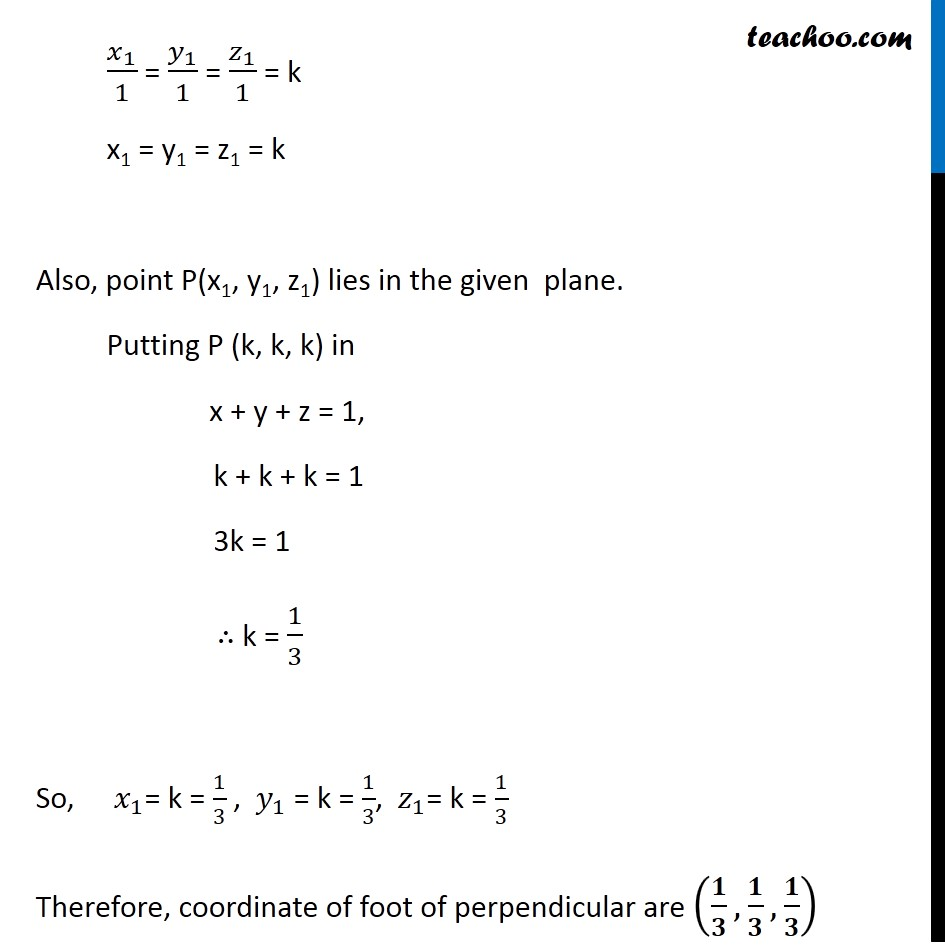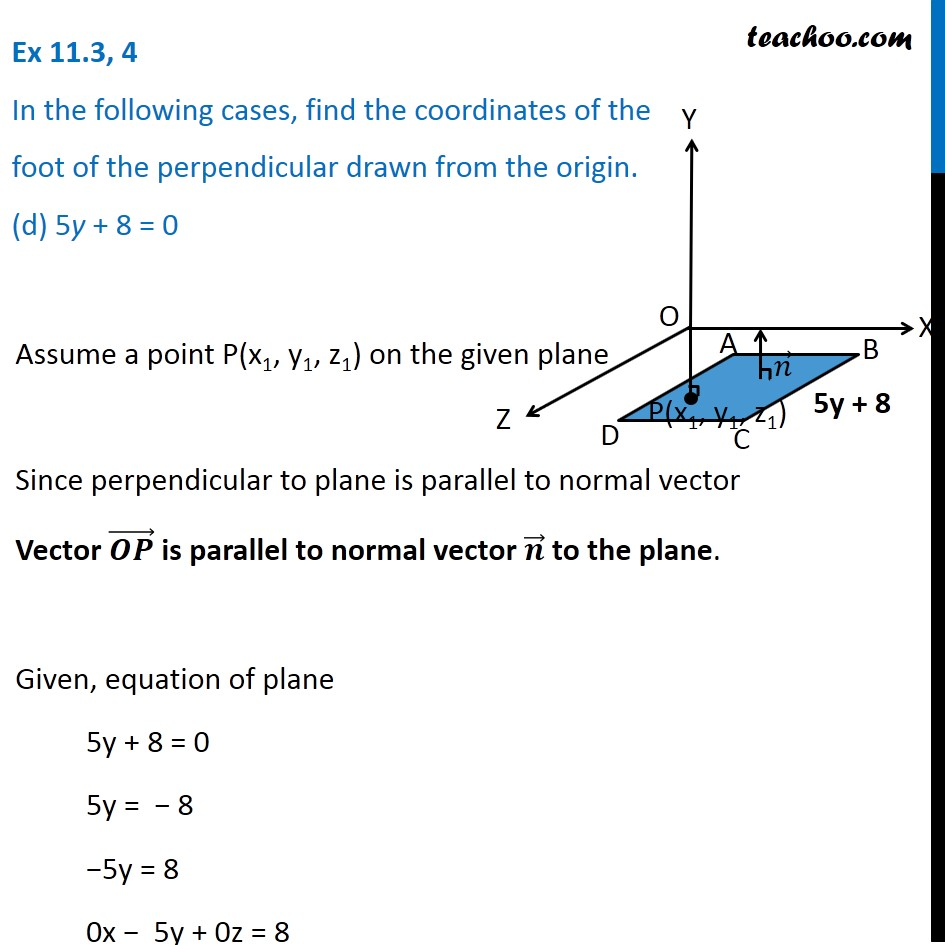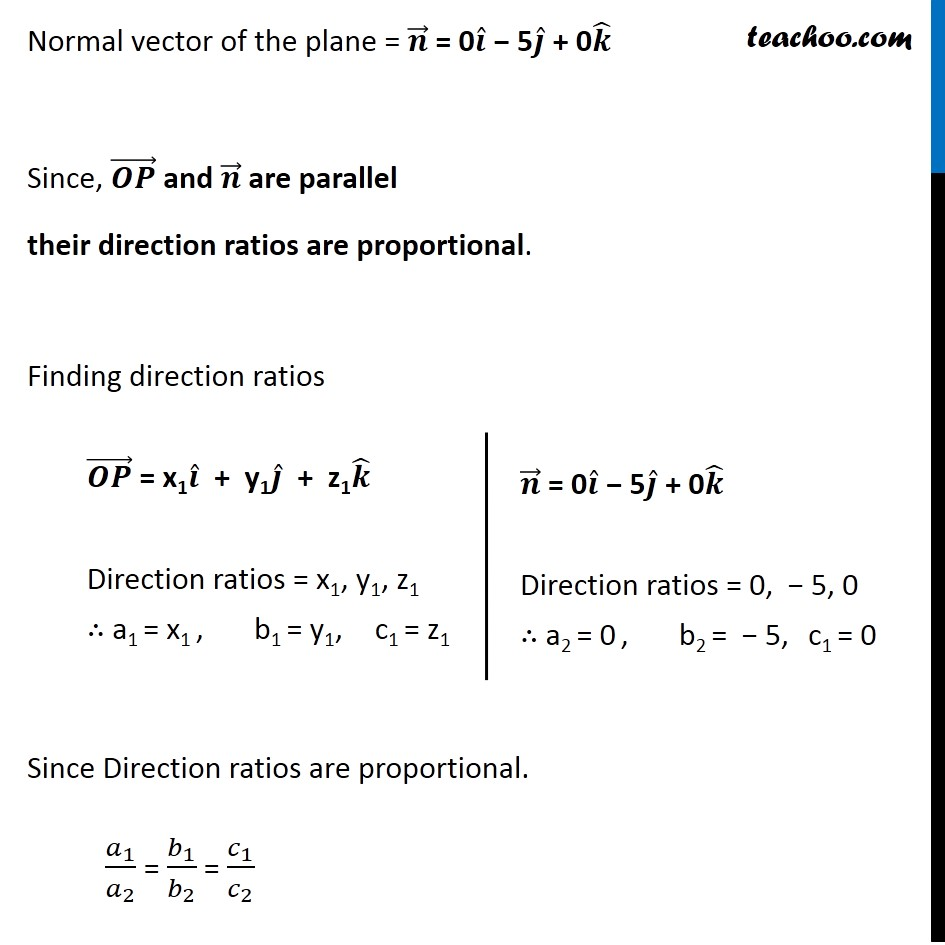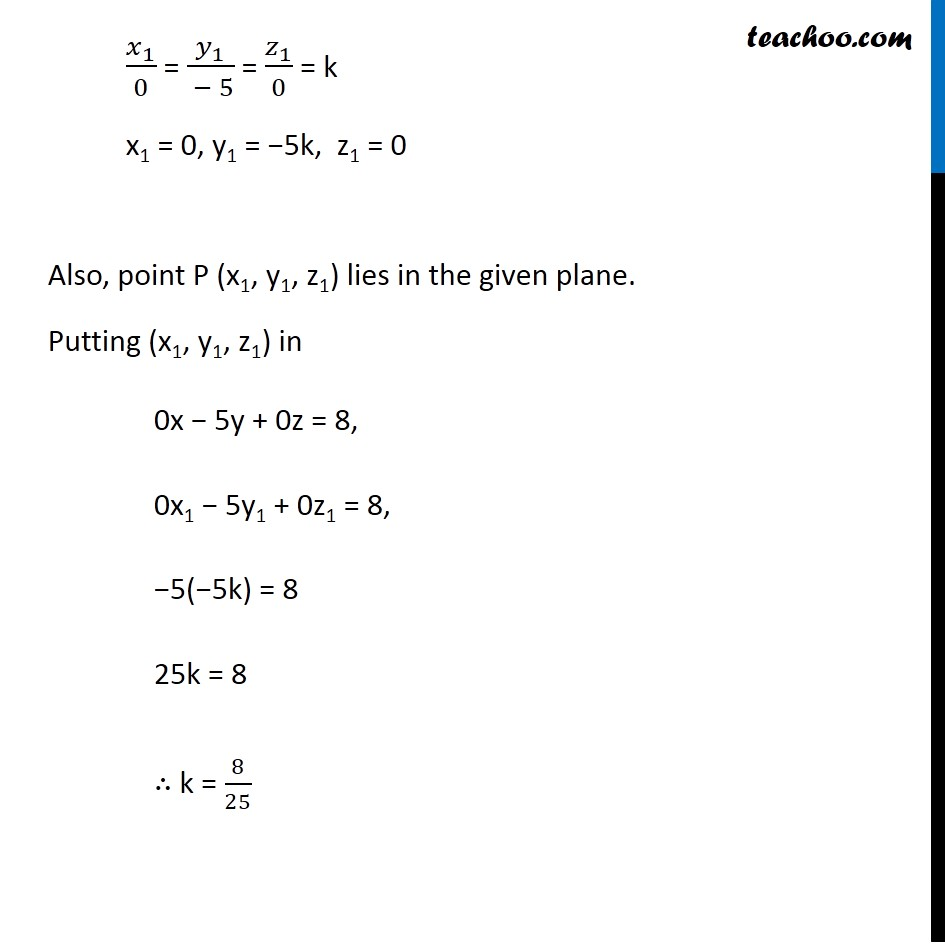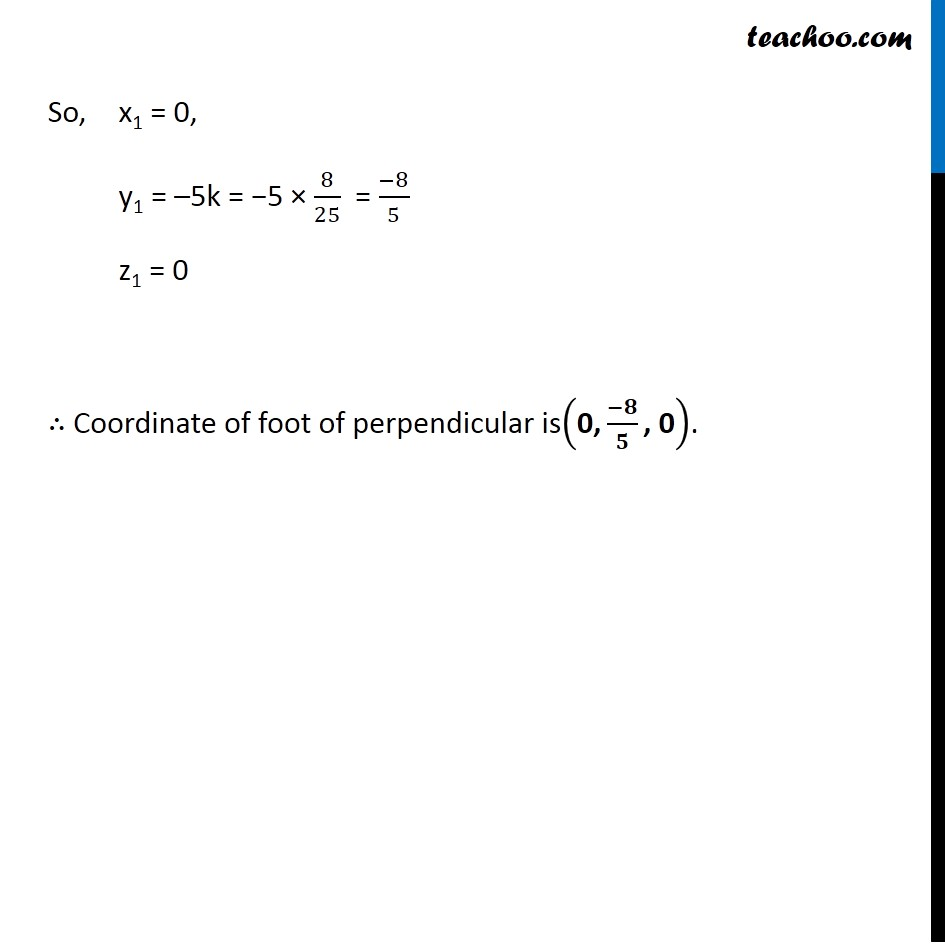Subscribe to our Youtube Channel - https://you.tube/teachoo

1. Chapter 11 Class 12 Three Dimensional Geometry
2. Serial order wise
3. Ex 11.3

Transcript

Ex 11.3, 4 In the following cases, find the coordinates of the foot of the perpendicular drawn from the origin. (a) 2x + 3y + 4z 12 = 0 Assume a point P(x1, y1, z1) on the given plane Since perpendicular to plane is parallel to normal vector Vector is parallel to normal vector to the plane. Given equation of plane is 2x + 3y + 4z 12 = 0 2x + 3y + 4z = 12 Since, and are parallel their direction ratios are proportional. Finding direction ratios Direction ratios are proportional So, 1 2 = 1 2 = 1 2 = k 1 2 = 1 3 = 1 4 = k x1 = 2k , y1 = 3k , z1 = 4k Also, point P(x1, y1, z1) lies in the plane. Putting P (2k, 3k, 4k) in 2x + 3y + 4z = 12, 2(2k) + 3(3k) + 4(4k) = 12 4k + 9k + 16k = 12 29k = 12 k = 12 29 So, 1 = 2k = 2 12 29 = 24 29 1 = 3k = 3 12 29 = 36 29 & 1 = 4k = 4 12 29 = 48 29 Therefore, coordinate of foot of perpendicular are , , Ex 11.3, 4 In the following cases, find the coordinates of the foot of the perpendicular drawn from the origin. (b) 3y + 4z 6 = 0 Assume a point P(x1, y1, z1) on the given plane Since perpendicular to plane is parallel to normal vector Vector is parallel to normal vector to the plane. Given equation of plane is 3y + 4z 6 = 0 3y + 4z = 6 0x + 3y + 4z = 6 Since, and are parallel their direction ratios are proportional. Finding direction ratios Direction ratios are proportional So, 1 2 = 1 2 = 1 2 = k 1 0 = 1 3 = 1 4 = k x1 = 0 , y1 = 3k , z1 = 4k Also, point P(x1, y1, z1) lies in the given plane. Putting P (0, 3k, 4k) in 0x + 3y + 4z = 6, 0 (k) + 3(3k) + 4(4k) = 6 25k = 6 k = 6 25 So, 1 = 0 1 = 3k = 3 6 25 = 18 25 1 = 4k = 4 6 25 = 24 25 Therefore, coordinates of foot of perpendicular are , , Ex 11.3, 4 In the following cases, find the coordinates of the foot of the perpendicular drawn from the origin. (c) x + y + z = 1 Assume a point P (x1, y1, z1) on the plane. Since perpendicular to plane is parallel to normal vector Vector is parallel to normal vector to the plane. Given, equation of the plane is x + y + z = 1 1x + 1y + 1z = 1 Since, and are parallel their direction ratios are proportional. Finding direction ratios Direction ratios are proportional So, 1 2 = 1 2 = 1 2 = k 1 1 = 1 1 = 1 1 = k x1 = y1 = z1 = k Also, point P(x1, y1, z1) lies in the given plane. Putting P (k, k, k) in x + y + z = 1, k + k + k = 1 3k = 1 k = 1 3 So, 1 = k = 1 3 , 1 = k = 1 3 , 1 = k = 1 3 Therefore, coordinate of foot of perpendicular are , , Ex 11.3, 4 In the following cases, find the coordinates of the foot of the perpendicular drawn from the origin. (d) 5y + 8 = 0 Assume a point P(x1, y1, z1) on the given plane Since perpendicular to plane is parallel to normal vector Vector is parallel to normal vector to the plane. Given, equation of plane 5y + 8 = 0 5y = 8 5y = 8 0x 5y + 0z = 8 Since, and are parallel their direction ratios are proportional. Finding direction ratios Since Direction ratios are proportional. 1 2 = 1 2 = 1 2 1 0 = 1 5 = 1 0 = k x1 = 0 , y1 = 5k z1 = 0 Also, point P (x1, y1, z1) lies in the given plane. Putting (x1, y1, z1) in 0x 5y + 0z = 8, 0x1 5y1 + 0z1 = 8, 5( 5k) = 8 25k = 8 k = 8 25 So, x1 = 0, y1 = 5k = 5 8 25 = 8 5 z1 = 0 Coordinate of foot of perpendicular is 0, , 0 .

Ex 11.3